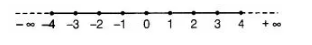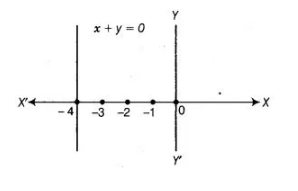# How many solutions) of the equation 2x + 1 = x – 3`
Question:

How many solutions) of the equation 2x + 1 = x – 3 are there on the

(ii) Cartesian plane?

Solution:

2x + 1 = x – 3

2x-x = -3-1

∴ x = – 4 ………..(i)

and it can be written as 1.x + 0. y = – 4 …………..(ii)

(i) Number line represent the all real values of x on the X-axis. Therefore, x = – 4 is exactly one point which lies on the number line.(ii) Whereas the equation x + 4 = 0 represent a straight line parallel to Y-axis and infinitely many points lie on a line in the cartesian plane.